International
Tables for
Crystallography
Volume D
Physical properties of crystals
Edited by A. Authier

International Tables for Crystallography (2006). Vol. D, ch. 1.4, pp. 99-100

## Section 1.4.1. Definition, symmetry and representation surfaces

H. Küppersa*

aInstitut für Geowissenshaften, Universität Kiel, Olshausenstrasse 40, D-24098 Kiel, Germany
Correspondence e-mail: kueppers@min.uni-kiel.de

### 1.4.1. Definition, symmetry and representation surfaces

| top | pdf |

If the temperature T of a solid is raised by an amount ΔT, a deformation takes place that is described by the strain tensor: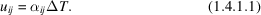The quantitiesare the coefficients of thermal expansion. They have dimensions of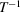and are usually given in units of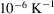. Sinceis a symmetrical polar tensor of second rank and T is a scalar,is a symmetrical polar tensor of second rank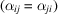. According to the properties of the strain tensor(cf. Section 1.3.1.3.2), the volume thermal expansion', β, is given by the (invariant) trace of the linear' coefficients.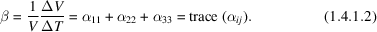The magnitudes of thermal expansion in different directions,, can be visualized in the following ways:

 (1) The representation quadric (cf. Section 1.1.3.5.2)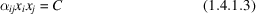can be transformed to principal axes,and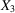with principal values,and: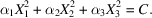The length of any radius vector leading to the surface of the quadricrepresents the reciprocal of the square root of thermal expansion along that direction,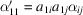(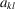are the direction cosines of the particular direction). If allare positive, the quadric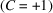is represented by an ellipsoid, whose semiaxes have lengths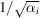. In this case, the square of the reciprocal length of radius vector r,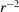, represents the amount of positive expansion in the particular direction, i.e. a dilation with increasing temperature. If allare negative, C is set to −1. Then, the quadric is again an ellipsoid, andrepresents a negative expansion, i.e. a contraction with increasing temperature. If thehave different signs, the quadric is a hyperboloid. The asymptotic cone represents directions along which no thermal expansion occurs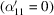. If one of theis negative, let us first choose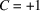. Then, the hyperboloid has one (belt-like) sheet (cf. Fig. 1.3.1.3) and the squares of reciprocal lengths of radius vectors leading to points on this sheet represent positive expansions (dilatations) along the particular directions. Along directions where the hyperboloid has no real values, negative expansions occur. To visualize these, C is set to −1. The resulting hyperboloid has two (cap-like) sheets (cf. Fig. 1.3.1.3) andrepresents the amount of contraction along the particular direction. If two of theare negative, the situation is complementary to the previous case. (2) A crystal sample having spherical shape (radius = 1 at temperature T) will change shape, after a temperature increase ΔT, to an ellipsoid with principal axes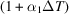,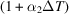and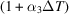. This `strain ellipsoid' is represented by the formula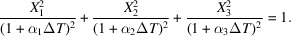Whereas the strain quadric (1.4.1.3)may be a real or imaginary ellipsoid or a hyperboloid, the strain ellipsoid is always a real ellipsoid. (3) The magnitude of thermal expansion in a certain direction (the longitudinal effect),, if plotted as radius vector, yields an oval: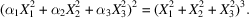If spherical coordinates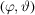are used to specify the direction, the length of r is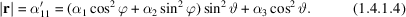Sections through this representation surface are called polar diagrams.

The three possible graphical representations are shown in Fig. 1.4.1.1.Figure 1.4.1.1 | top | pdf |Sections (ac plane) of representation surfaces for a trigonal (or tetragonal or hexagonal) crystal with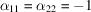and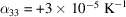(similar to calcite). (a) Quadric, (b) strain ellipsoid (greatly exaggerated), (c) polar diagram. The c axis is the axis of revolution. Sectors with negative expansions are dashed.

The maximum number of independent components of the tensoris six (in the triclinic system). With increasing symmetry, this number decreases as described in Chapter 1.1. Accordingly, the directions and lengths of the principal axes of the representation surfaces are restricted as described in Chapter 1.3(e.g. in hexagonal, trigonal and tetragonal crystals, the representation surfaces are rotational sheets and the rotation axis is parallel to the n-fold axis). The essential results of these symmetry considerations, as deduced in Chapter 1.1and relevant for thermal expansion, are compiled in Table 1.4.1.1.

 Table 1.4.1.1| top | pdf | Shape of the quadric and symmetry restrictions
SystemQuadricNo. of independent componentsNonzero components
ShapeDirection of principal axes
Triclinic General ellipsoid or hyperboloid No restrictions 6Monoclinic One axis parallel to twofold axis (b) 4Orthorhombic Parallel to crystallographic axes 3Trigonal, tetragonal, hexagonal Revolution ellipsoid or hyperboloid c axis is revolution axis 2Cubic, isotropic media Sphere Arbitrary, not defined 1The coefficients of thermal expansion depend on temperature. Therefore, the directions of the principal axes of the quadrics in triclinic and monoclinic crystals change with temperature (except the principal axis parallel to the twofold axis in monoclinic crystals).

The thermal expansion of a polycrystalline material can be approximately calculated if thetensor of the single crystal is known. Assuming that the grains are small and of comparable size, and that the orientations of the crystallites are randomly distributed, the following average of[(1.4.1.4)] can be calculated: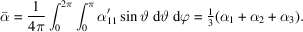If the polycrystal consists of different phases, a similar procedure can be performed if the contribution of each phase is considered with an appropriate weight.

It should be mentioned that the true situation is more complicated. The grain boundaries of anisotropic polycrystalline solids are subject to considerable stresses because the neighbouring grains have different amounts of expansion or contraction. These stresses may cause local plastic deformation and cracks may open up between or within the grains. These phenomena can lead to a hysteresis behaviour when the sample is heated up or cooled down. Of course, in polycrystals of a cubic crystal species, these problems do not occur.

If the polycrystalline sample exhibits a texture, the orientation distribution function (ODF) has to be considered in the averaging process. The resulting overall symmetry of a textured polycrystal is usually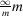(see Section 1.1.4.7.4.2), showing the same tensor form as hexagonal crystals (Table 1.4.1.1), or mmm.